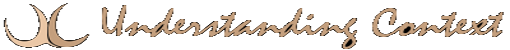# 27 Mar statistical analysis

A process of assembling results and applying various mathematical formulae to analyze characteristics and patterns in the results. Stochastic techniques and probability theory provide tools for statistical analysis, as do the standard methods of calculating mean, median, average and standard deviation. The results of statistical analyses can often be depicted graphically, making them more meaningful.
Keywords: stochastic
probability
analysis
understanding
inference
standard deviation
range
References: algorithms
automata
computing
formalisms
logic
rules

This site uses Akismet to reduce spam. Learn how your comment data is processed.